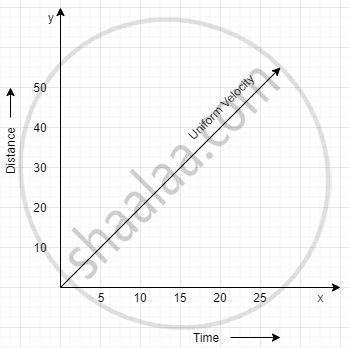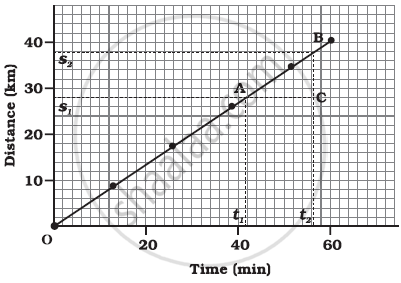Advertisement Remove all ads

# Graphical Representation of Linear Motion - Distance - Time Graphs

Advertisement Remove all ads

#### notes

Graphical representation of motion- Distance-Time Graphs:Calculation Of Velocity By Using Distance Time Graph:To calculate the velocity, let take two points A and B on the slope OB.

Draw one line parallel to y-axis and another parallel to x-axis from B.

Again draw a line parallel to y-axis and another parallel to x-axis from point A.

Let, line parallel to x-axis from point B cut at a point, S2 at y-axis.

Line parallel to x-axis from point A cut at point, S1 at y-axis.

Let, line parallel to y-axis from point B cut at t2 at x-axis.

Line parallel to y-axis from point A cut at t1 at x-axis.

Now, BC= Distance = S2 – S1 and AC = time = t2 – t1

We know that slope of the graph is given by the ratio of change in y-axis and change in X-axis.

Or, "Slope" = "Change in y-axis" / "Change in x-axis"

Thus, "slope, AB"="BC"/"AC"

Or, "v"="s2−s1"/"t2−t1"

Where, v= velocity, (s2−s1) = interval of distance and (t2−t1)= time interval

Thus, "Velocity"="Distance" / "Time"

If you would like to contribute notes or other learning material, please submit them using the button below.

### Shaalaa.com

Motion part 10 (Graphical representation of motion) [00:08:32]
S
0%

#### Related concepts

Advertisement Remove all ads
Share
Notifications

View all notifications

Forgot password?
Course